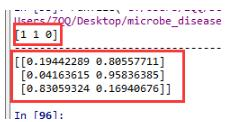# 关于Python sklearn中的.fit与.predict的用法

```clf=KMeans(n_clusters=5) #创建分类器对象
fit_clf=clf.fit(X) #用训练器数据拟合分类器模型
clf.predict(X) #也可以给新数据数据对其预测

print(clf.cluster_centers_) #输出5个类的聚类中心

y_pred = clf.fit_predict(X) #用训练器数据X拟合分类器模型并对训练器数据X进行预测

print(y_pred) #输出预测结果```

model.predict_proba(x)不同于model.predict()，它返回的预测值为获得所有结果的概率。（有多少个分类结果，每行就有多少个概率，对每个结果都有一个概率值，如0、1两分类就有两个概率）

python3 代码实现：

```# -*- coding: utf-8 -*-
"""
Created on Sat Jul 27 21:25:39 2019

@author: ZQQ
"""
from sklearn.linear_model import LogisticRegression
from sklearn.ensemble import RandomForestClassifier
from xgboost import XGBClassifier
import numpy as np
import warnings
warnings.filterwarnings("ignore")
# 这个方法只是解决了表面，没有根治

# 数据（特征，属性）
x_train = np.array([[1,2,3],
[1,5,4],
[2,2,2],
[4,5,6],
[3,5,4],
[1,7,2]])
# 数据的标签
y_train = np.array([1, 0, 1, 1, 0, 0])

# 测试数据
x_test = np.array([[2,1,2],
[3,2,6],
[2,6,4]])

# 导入模型
model = LogisticRegression()

#model = RandomForestClassifier()

#model=XGBClassifier()

model.fit(x_train, y_train)

# 返回预测标签
print(model.predict(x_test))

print('---------------------------------------')

# 返回预测属于某标签的概率
print(model.predict_proba(x_test))

```